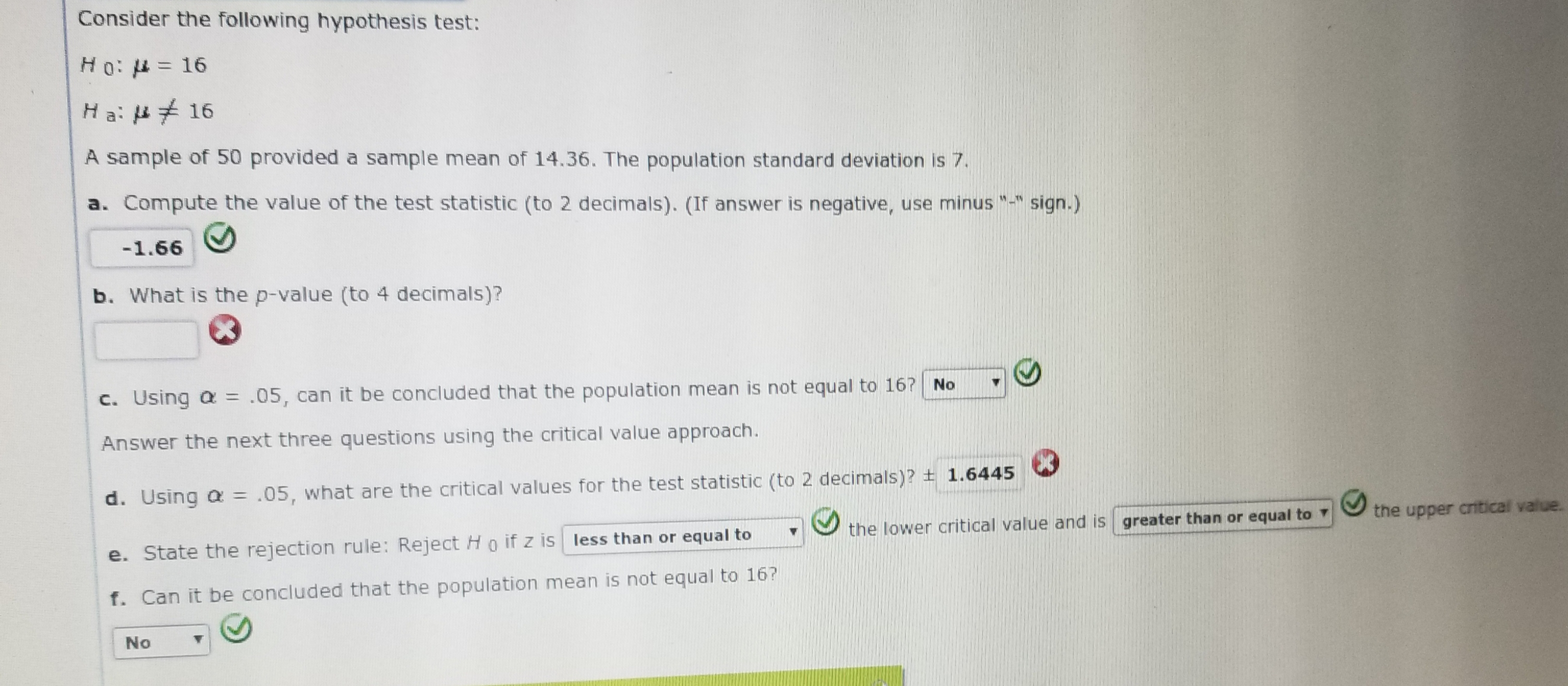Consider the following hypothesis test:H 0: μ-16Ha: μ 16A sample of 50 provided a sample mean of 14.36. The population standard deviation is 7.a. Compute the value of the test statistic (to 2 decimals). (If answer is negative, use minussign.)b. What is the p-value (to 4 decimals)?c. Using α .05, can it be concluded that the population mean is not equal to 1671 No-Answer the next three questions using the critical value approach.d, using α = .05, what are the critical values for the test statistic (to 2 decimals)?土1.6445 \e. State the rejection rule: Reject H o f z is less than or equal tothe lower critical value and is areater than or squal tothe upper anticalf. Can it be concluded that the population mean is not equal to 16No

Questionhelp_outlineImage TranscriptioncloseConsider the following hypothesis test: H 0: μ-16 Ha: μ 16 A sample of 50 provided a sample mean of 14.36. The population standard deviation is 7. a. Compute the value of the test statistic (to 2 decimals). (If answer is negative, use minus sign.) b. What is the p-value (to 4 decimals)? c. Using α .05, can it be concluded that the population mean is not equal to 1671 No- Answer the next three questions using the critical value approach. d, using α = .05, what are the critical values for the test statistic (to 2 decimals)?土1.6445 \ e. State the rejection rule: Reject H o f z is less than or equal tothe lower critical value and is areater than or squal tothe upper antical f. Can it be concluded that the population mean is not equal to 16 No fullscreen
Step 1

Given a two tail hypothesis test

Null Hypothesis : µ = 16

Alternative Hypothesis : µ ≠ 16

Sample mean = 14.36 and stadnard deviation 7 with sample size n = 50 test statistic is calculated an...

Want to see the full answer?

See Solution

Want to see this answer and more?

Our solutions are written by experts, many with advanced degrees, and available 24/7

See Solution
Tagged in

Statistics